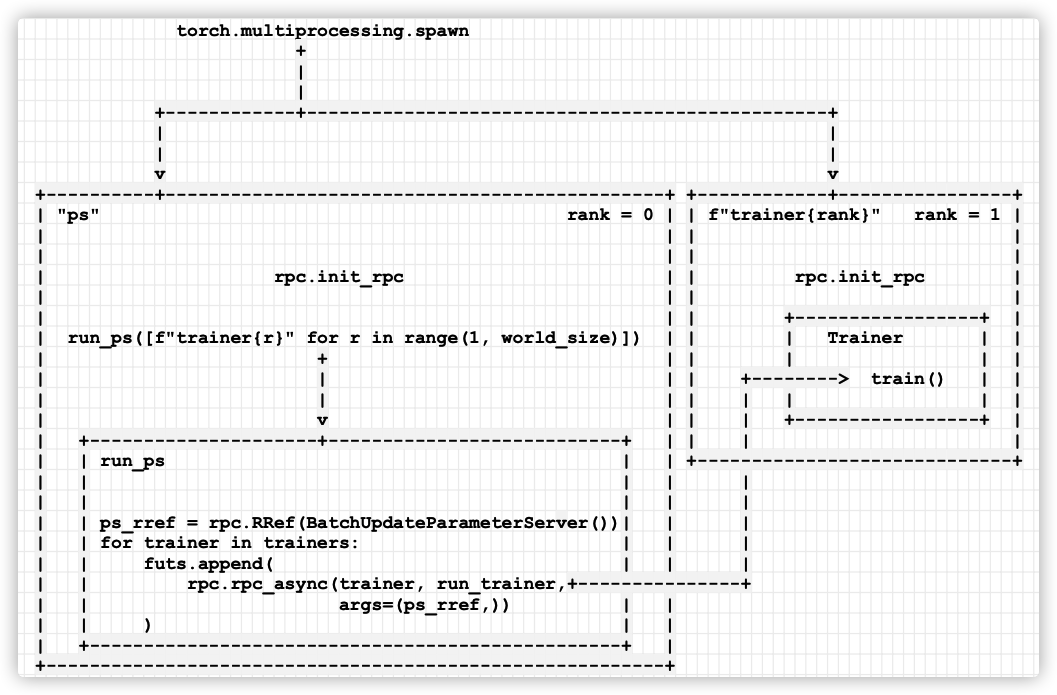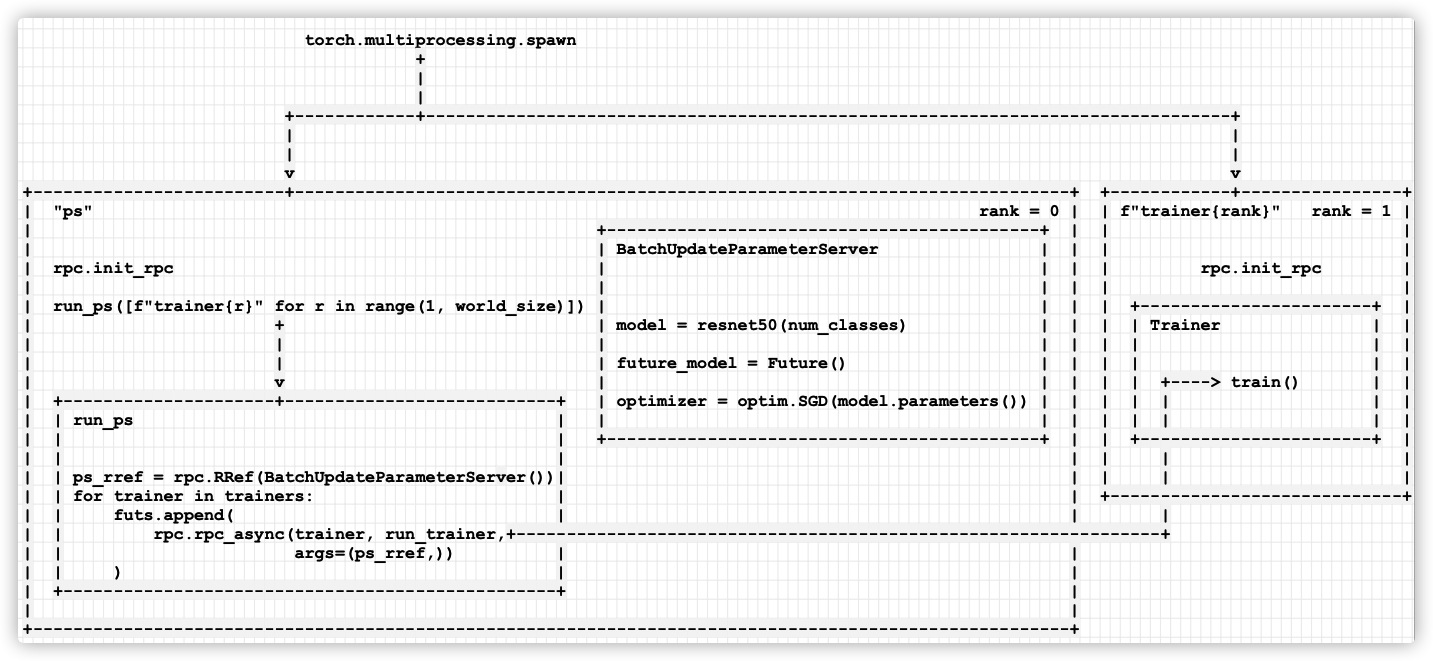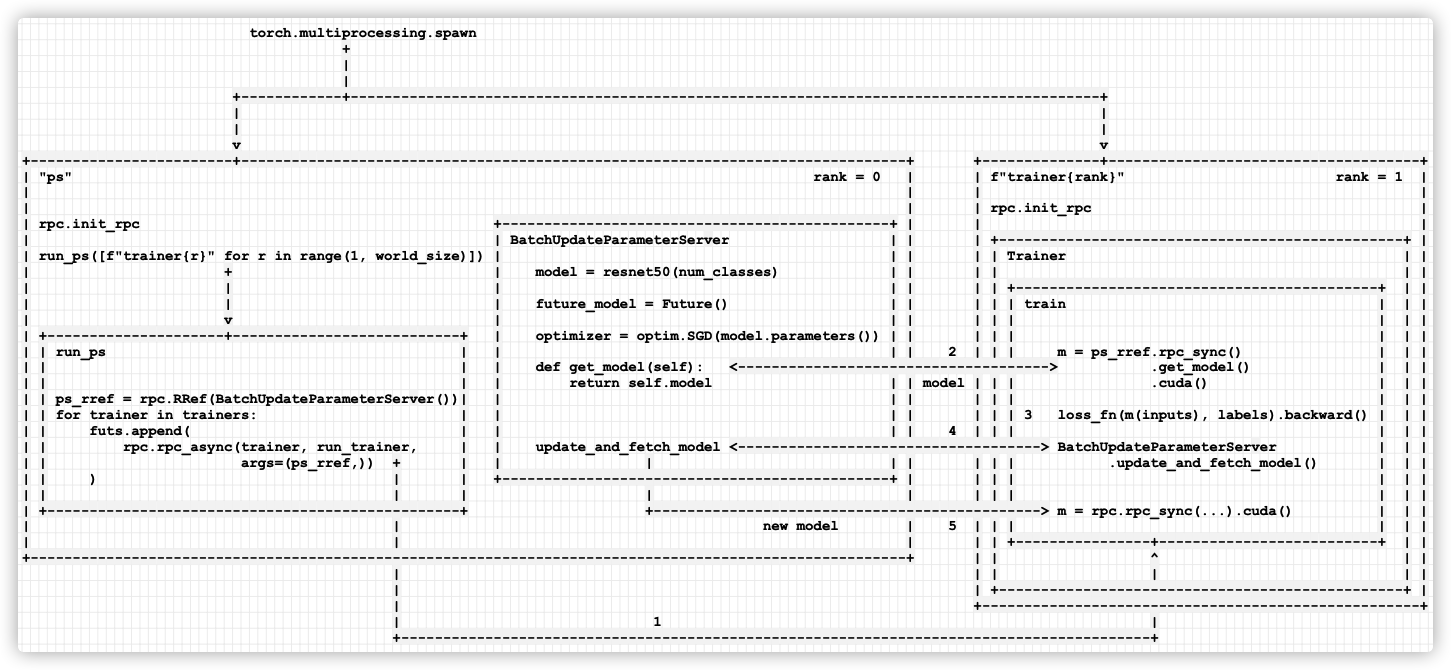# [源码解析] PyTorch 分布式(16) --- 使用异步执行实现批处理 RPC

## 0x00 摘要

PyTorch分布式其他文章如下：

## 0x01 前言

### 1.2 基础知识

• torch.futures.Future 封装了一个异步执行，同时也支持安装回调函数。
• @rpc.functions.async_execution 装饰器，它允许应用程序告诉被调用者，本目标函数将返回一个future，并且可以在执行过程中多次暂停和yield。

### 1.3 代码

import os
from datetime import datetime

import torch
import torch.distributed.rpc as rpc
import torch.multiprocessing as mp
import torch.nn as nn
from torch import optim

import torchvision

batch_size = 20
image_w = 64
image_h = 64
num_classes = 30
batch_update_size = 5
num_batches = 6

def timed_log(text):
print(f"{datetime.now().strftime('%H:%M:%S')} {text}")

class BatchUpdateParameterServer(object):

def __init__(self, batch_update_size=batch_update_size):
self.model = torchvision.models.resnet50(num_classes=num_classes)
self.future_model = torch.futures.Future()
self.batch_update_size = batch_update_size
self.curr_update_size = 0
self.optimizer = optim.SGD(self.model.parameters(), lr=0.001, momentum=0.9)
for p in self.model.parameters():

def get_model(self):
return self.model

@staticmethod
@rpc.functions.async_execution
self = ps_rref.local_value()
for p, g in zip(self.model.parameters(), grads):
with self.lock:
self.curr_update_size += 1
fut = self.future_model

if self.curr_update_size >= self.batch_update_size:
for p in self.model.parameters():
self.curr_update_size = 0
self.optimizer.step()
fut.set_result(self.model)
timed_log("PS updated model")
self.future_model = torch.futures.Future()

return fut

class Trainer(object):

def __init__(self, ps_rref):
self.ps_rref = ps_rref
self.loss_fn = nn.MSELoss()
self.one_hot_indices = torch.LongTensor(batch_size) \
.random_(0, num_classes) \
.view(batch_size, 1)

def get_next_batch(self):
for _ in range(num_batches):
inputs = torch.randn(batch_size, 3, image_w, image_h)
labels = torch.zeros(batch_size, num_classes) \
.scatter_(1, self.one_hot_indices, 1)
yield inputs.cuda(), labels.cuda()

def train(self):
name = rpc.get_worker_info().name
m = self.ps_rref.rpc_sync().get_model().cuda()
for inputs, labels in self.get_next_batch():
timed_log(f"{name} processing one batch")
self.loss_fn(m(inputs), labels).backward()
m = rpc.rpc_sync(
self.ps_rref.owner(),
BatchUpdateParameterServer.update_and_fetch_model,
args=(self.ps_rref, [p.grad for p in m.cpu().parameters()]),
).cuda()
timed_log(f"{name} got updated model")

def run_trainer(ps_rref):
trainer = Trainer(ps_rref)
trainer.train()

def run_ps(trainers):
timed_log("Start training")
ps_rref = rpc.RRef(BatchUpdateParameterServer())
futs = []
for trainer in trainers:
futs.append(
rpc.rpc_async(trainer, run_trainer, args=(ps_rref,))
)

torch.futures.wait_all(futs)
timed_log("Finish training")

def run(rank, world_size):
os.environ['MASTER_PORT'] = '29500'
options=rpc.TensorPipeRpcBackendOptions(
rpc_timeout=0  # infinite timeout
)
if rank != 0:
rpc.init_rpc(
f"trainer{rank}",
rank=rank,
world_size=world_size,
rpc_backend_options=options
)
# trainer passively waiting for ps to kick off training iterations
else:
rpc.init_rpc(
"ps",
rank=rank,
world_size=world_size,
rpc_backend_options=options
)
run_ps([f"trainer{r}" for r in range(1, world_size)])

# block until all rpcs finish
rpc.shutdown()

if __name__=="__main__":
world_size = batch_update_size + 1
mp.spawn(run, args=(world_size, ), nprocs=world_size, join=True)


## 0x02 启动

### 2.1 总体启动

def run(rank, world_size):
os.environ['MASTER_PORT'] = '29500'
options=rpc.TensorPipeRpcBackendOptions(
rpc_timeout=0  # infinite timeout
)
if rank != 0:
rpc.init_rpc( # 训练代码
f"trainer{rank}",
rank=rank,
world_size=world_size,
rpc_backend_options=options
)
# trainer passively waiting for ps to kick off training iterations
else:
rpc.init_rpc( # 参数服务器
"ps",
rank=rank,
world_size=world_size,
rpc_backend_options=options
)
run_ps([f"trainer{r}" for r in range(1, world_size)])

# block until all rpcs finish
rpc.shutdown()

if __name__=="__main__":
world_size = batch_update_size + 1
mp.spawn(run, args=(world_size, ), nprocs=world_size, join=True)


             torch.multiprocessing.spawn
+
|
|
+------------+-------------------------------------------------
|                                                             |
|                                                             |
v                                                             v
+----------+----------------------------------------------+ +------------+----------------+
| "ps"                                           rank = 0 | | f"trainer{rank}"   rank = 1 |
|                                                         | |                             |
|                                                         | |                             |
|                     rpc.init_rpc                        | |         rpc.init_rpc        |
|                                                         | |                             |
|                                                         | |                             |
|  run_ps([f"trainer{r}" for r in range(1, world_size)])  | |                             |
|                                                         | |                             |
|                                                         | |                             |
+---------------------------------------------------------+ +-----------------------------+



### 2.2 启动参数服务器

run_ps 启动了参数服务器和trainer。注意，这里在参数服务器之中启动 trainer，即，master 不仅仅有一个参数服务器，还负责通过 rpc 来驱动trainer上的训练循环。

def run_ps(trainers):
timed_log("Start training")
ps_rref = rpc.RRef(BatchUpdateParameterServer())
futs = []
for trainer in trainers: # trainer 是字符串，比如"trainer1"
futs.append(
rpc.rpc_async(trainer, run_trainer, args=(ps_rref,)) # 运行run_trainer
)

torch.futures.wait_all(futs)
timed_log("Finish training")

def run_trainer(ps_rref):
trainer = Trainer(ps_rref)
trainer.train() # 调用 Trainer 的方法## 0x03 参数服务器

• PS初始化时候生成了常规SGB优化器，不是分布式优化器，而且优化器是在PS之上
• update_and_fetch_model方法被 @rpc.functions.async_execution所装饰，将由trainer调用。
• 每次调用都会返回一个Future对象，该对象将被用来处理更新后的模型。
• 大多数训练器发起的调用只是累积梯度到 .grad成员变量 ，然后立即返回，并在 PS 上产生 RPC 线程。
• 最后到达的训练器将触发优化器步骤并消耗所有先前上报的梯度。然后它使用更新后的模型来设置future_model，这是依靠通过Future对象来依次通知来自其他训练者的先前请求，并将更新后的模型发送给所有训练者。

batch_size = 20
image_w = 64
image_h = 64
num_classes = 30
batch_update_size = 5
num_batches = 6

def timed_log(text):
print(f"{datetime.now().strftime('%H:%M:%S')} {text}")

class BatchUpdateParameterServer(object):

def __init__(self, batch_update_size=batch_update_size):
self.model = torchvision.models.resnet50(num_classes=num_classes)
self.future_model = torch.futures.Future()
self.batch_update_size = batch_update_size
self.curr_update_size = 0
# 重点：这里是常规SGB优化器，不是分布式优化器
self.optimizer = optim.SGD(self.model.parameters(), lr=0.001, momentum=0.9)
for p in self.model.parameters():

def get_model(self):
return self.model

@staticmethod
@rpc.functions.async_execution # trainer会直接调用
self = ps_rref.local_value()
for p, g in zip(self.model.parameters(), grads): # 得到
with self.lock:
self.curr_update_size += 1
fut = self.future_model

if self.curr_update_size >= self.batch_update_size:
# 最后到达的训练器将触发优化器步骤并消耗所有先前上报的梯度。
for p in self.model.parameters():
self.curr_update_size = 0
self.optimizer.step() # 更新模型
fut.set_result(self.model) # 将更新后的模型发送给所有训练者
timed_log("PS updated model")
self.future_model = torch.futures.Future() # 使用更新后的模型来设置future_model

return fut # 该对象将被用来处理更新后的模型## 0x04 Trainer

• 每个训练器首先运行前向和后向传播以在本地生成梯度。
• 然后，每个训练器使用 RPC 向 PS 报告其梯度，并通过同一 RPC 请求的返回值取回更新后的参数。

class Trainer(object):

def __init__(self, ps_rref):
self.ps_rref = ps_rref
self.loss_fn = nn.MSELoss()
self.one_hot_indices = torch.LongTensor(batch_size) \
.random_(0, num_classes) \
.view(batch_size, 1)

def get_next_batch(self):
for _ in range(num_batches):
inputs = torch.randn(batch_size, 3, image_w, image_h)
labels = torch.zeros(batch_size, num_classes) \
.scatter_(1, self.one_hot_indices, 1)
yield inputs.cuda(), labels.cuda()

def train(self):
name = rpc.get_worker_info().name
# 从参数服务器获取模型
m = self.ps_rref.rpc_sync().get_model().cuda()
for inputs, labels in self.get_next_batch():
timed_log(f"{name} processing one batch")
# 利用模型来前向传播/反向传播
self.loss_fn(m(inputs), labels).backward()
# 调用参数服务器的函数来提交梯度
m = rpc.rpc_sync( # rpc_sync 操作完成之后，m就是最新模型了
self.ps_rref.owner(),
BatchUpdateParameterServer.update_and_fetch_model,
args=(self.ps_rref, [p.grad for p in m.cpu().parameters()]),
).cuda()
timed_log(f"{name} got updated model")


1. 参数服务器的run_trainer 方法会直接调用 trainer.train() 方法来执行一步step。
2. train 方法之中，会调用 self.ps_rref.rpc_sync().get_model().cuda() 从参数服务器获得模型，放到本地设备之上（图上是双向箭头，表示这是一个get/return动作，需要把模型存储在worker本地）。
3. 调用 self.loss_fn(m(inputs), labels).backward() 来进行前向传播/反向传播。
4. 调用参数服务器的 update_and_fetch_model 函数来提交梯度，这里使用了异步RPC
5. 参数服务器的 update_and_fetch_model 之中，进行梯度累积，模型更新是通过PS之上常规SGD优化器完成，最后调用 fut.set_result(self.model) 来发布新模型给trainer。在trainer 之中，就是 m = rpc.rpc_sync(...) 这个赋值之后，m 是最新模型了。## 0x05 对比

• ps-lite 是类似传统服务器实现，有自己主动的业务循环，可以响应用户的显式请求，也有自己明确的逻辑，本地也有自己的KV存储。
• PyTorch 前两篇官方文档（本系列前两篇文章）之中，参数服务器则是另外一种思路：
• 参数服务器上没有主动的循环，没有KV存储，没有服务器逻辑，而是可以直接存储业务模型，ps 会把业务模型需要优化的参数返回给trainer 之上的 DistributedOptimizer。
• 业务驱动由trainer完成：train loop代码在trainer 之中，DistributedOptimizer 在trainer 之中，DistributedOptimizer 负责进行分布式优化。
• 本文又与上面不同，看起来更像是ps-lite，但是又糅合了RPC实现：
• ps进程会启动trainer的训练循环
• 每个迭代之中，trainer 会从参数服务器获取最新模型，前向操作/后向传播都在trainer 完成。
• trainer 会通过异步RPC把梯度提交给参数服务器。
• 模型更新是通过PS之上常规SGD优化器完成
• 模型更新之后通过异步RPC把模型再次分发给trainer。

## 0xFF 参考

IMPLEMENTING BATCH RPC PROCESSING USING ASYNCHRONOUS EXECUTIONS

posted @ 2021-12-15 21:04  罗西的思考  阅读(236)  评论(0编辑  收藏  举报